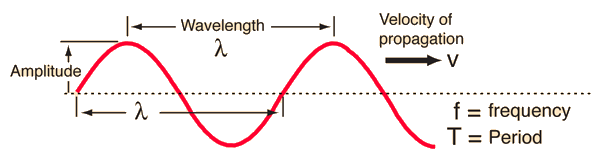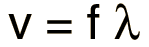# Traveling Wave Relationship

A single frequency traveling wave will take the form of a sine wave. A snapshot of the wave in space at an instant of time can be used to show the relationship of the wave properties frequency, wavelength and propagation velocity.The motion relationship "distance = velocity x time" is the key to the basic wave relationship. With the wavelength as distance, this relationship becomes λ = vT. Then using f=1/T gives the standard wave relationshipCalculation

This is a general wave relationship which applies to sound and light waves, other electromagnetic waves, and waves in mechanical media.

 Wave plot example
Index

Traveling wave concepts

Periodic motion concepts

 HyperPhysics***** Sound R Nave
Go Back

# Wave Calculation

This is a general exploration calculation using the wave relationship.## Wave velocity = frequency x wavelength

### Wavelength = m = x10^m = x10^ft. Frequency = Hz = x10^Hz Wave velocity = m/s =x10^m/s = x10^ft/s.

Any of the numbers may be changed. When you have finished entering data, click the active text above for the quantity you wish to calculate. Unit conversions will be calculated as you enter data, but the three wave parameters will not be forced to be consistent until you click on the desired quantity.
Caution: for very large values, the first value for each quantity may not display all the digits. The values in scientific notation should be used.
 Wave plot example
 Speed of sound in air
Index

Traveling wave concepts

Periodic motion concepts

 HyperPhysics***** Sound R Nave
Go Back